# Refractometry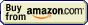## Refractometry » Snell's law

Refraction was researched by many scientists throughout the ages, and law describing the refraction was discovered separately several times only to be later forgotten. Now it is named after a Dutch astronomer and mathematician, Willebrord Snellius, although - for historical reasons - in some countries it is known as Snell-Descartes law (or even just Descartes law).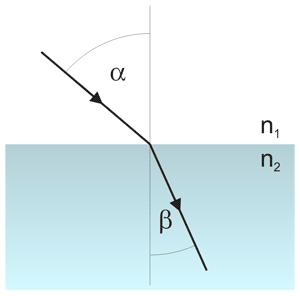Light refraction - incident (α) and refracted (β) rays, at the boundary between n1 and n2 media.

Snellius collected data on incident and refracted angles for many substances and found an equation that describes refraction on the boundary between them: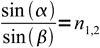1

This equation requires - for each pair of substances - experimental value describing the refractive property of the boundary (denoted here as n1,2). Analyzing the data Snellius found something more - if he knew characteristic constant for boundary of substances A and B (say nA,B), and boundary of substances B and C (nB,C), he could easily calculate characteristic constant for boundary between substances A and C - and it was just equal to the product of known constants: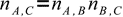2

That meant he didn't need a specific constant for each pair of substances, rather he could assing a single number to each substance, call it a refractive index - and use it to calculate characteristic of each boundary.

There is one problem left - to calculate all values he had to select one of the substances and assign an arbitrary value to it. He had chosen vacuum and assigned 1 to it, which meant all other values were always larger than 1 (we now know materials for which it is not necesarilly true). Now, his law took the form in which it is used up to today: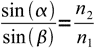3

where both indices can be taken from refractive indices table.

Snell's law is not limited to light, but describes refraction of any waves travelling through any media.

We should also not forget about fact that (as it was explained on the refraction page) refracted angle depends on the light speed, which is in turn function of wavelength - so values of indices are a function of wavelength as well.

This site is in construction. More to come. Page was last modified on March 17 2011, 00:00:01.